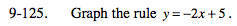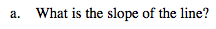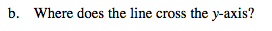### Home > MC2 > Chapter 9 > Lesson 9.3.4 > Problem9-125

9-125.Pick two points on the line and find the change in y-values over the change in x-values to find the slope.
What do you get? Does this value correspond with a value in the rule above?Where on the graph does the line cross the y-axis?
Also, logically, the graph will cross the y-axis when x = 0.
Using the rule, can you calculate this value?
What do you get? Does this value correspond with a value in the rule above?

(0, 5)